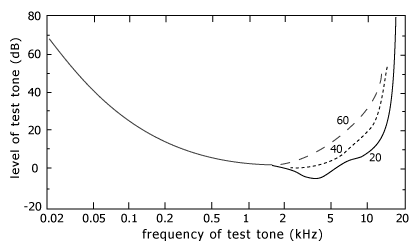## IB Physics Glossary

 Search full text

Browse the glossary using this index

Special | A | B | C | D | E | F | G | H | I | J | K | L | M | N | O | P | Q | R | S | T | U | V | W | X | Y | Z | ALL

### T

#### Temperature

absolute, is proportional to the average kinetic energy of the molecules of a substance. (d)
 Keyword(s): 3.1

#### The photoelectric effect

is the phenomena by which electrons are emitted from the surface of a metal being illuminated with light/electromagnetic radiation.

When light of different frequencies and different intensities is incident on the surface:

I. There exists a frequency of light (the threshold frequency) below which no electrons are emitted whatever the intensity of the light. Energy is needed to eject the electrons from the surface.

Light in this case is not behaving as a wave but as a particle (photon) since according to the wave model, the energy of a wave depends on its amplitude/intensity so one would expect emission to depend on intensity not frequency.

II. For light above the threshold frequency, the emission of the electrons is instantaneous whatever the intensity of the light.

Light in this case is not behaving as a wave but as a particle (photon) since according to the wave model, energy is delivered continuously to the surface so with a very low intensity wave one would expect the electrons to need a certain amount of time to gain sufficient energy to leave the surface.

$$E = hf = E_K + \phi$$

where E = h f is the photon energy, EK is the maximum kinetic energy of the photoelectrons and φ is the work function of the metal.

We can also write

$$E_K = hf - \phi$$

or with the Milikan experiment , EK = eVs, where Vs is the stopping potential

$$eV_s = hf - \phi$$

or

$$V_s = (\frac{h}{e})f - (\frac{\phi}{e})$$

A Vs-f graph yields a straight with gradient = $$(\frac{h}{e})$$ and y-intercept=$$(\frac{\phi}{e})$$.

Or

$$V_s = \frac{hc}{e} \times \frac{1}{\lambda} - \frac{\phi}{e}$$

A Vs-1/λ graph yields a straight with gradient = $$(\frac{hc}{e})$$ and y-intercept=$$(\frac{\phi}{e})$$.

Notes:

Consider increasing the frequency of light falling on a metallic surface while keeping the light intensity fixed. A greater stopping potential is required to reduce the photo-current to zero. Since the energy per photon increases but the same light intensity should be maintained, the number of actual number of photons, or the photon flux is less, therefore a smaller photo-current flows.

 Keyword(s): 13.1

#### Thermal/Heat Capacity

is the quantity of thermal energy required to raise the temperature of an object by one degree. (d)
 Keyword(s): 3.2

#### Threshold hearing curve

shows that the sound intensity required to be heard is quite different for different frequencies. Most sensitive frequencies (≈ 3kHz) have lower dB values - less sound intensity is required to for the sound to be heard. Least sensitive frequencies have higher dB values - more sound intensity is required for the sound to be heard. Negative dB values indicate sound intensity is below I0, the threshold intensity of hearing. The graph below shows how the curve varies with age: 20, 40 and 60 years.Keyword(s): I1

#### Threshold intensity of hearing

is the minimum intensity at which sound is heard, I0 = 1 x 10−12 Wm−2.
 Keyword(s): I1

#### Tinnitus

is the sensation of ringing in the ears which can be caused by short-term exposure to loud sounds.
 Keyword(s): I1

#### Translational equilibrium

occurs when there is no resultant force on an object in any direction:

$$\Sigma F = 0$$ which leads to $$a = 0 \rightarrow \Delta v = 0 \rightarrow v = \text{constant}$$.
 Keyword(s): 2.2